﻿ 用于枸杞有机肥深施的作业机械设计 Mechanical Design for Chinese Wolfberry Organic Fertilizer Deep Homework

Mechanical Engineering and Technology
Vol.07 No.01(2018), Article ID:23934,10 pages
10.12677/MET.2018.71009

Mechanical Design for Chinese Wolfberry Organic Fertilizer Deep Homework

Xu Ding1,2, Zhengyong Zhang1*, Yang Liu1, Yao Peng1,2

1Chinese Academy of Science, Hefei Institute of Intelligent Machines, Hefei Anhui

2University of Science and Technology of China, Hefei Anhui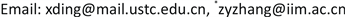Received: Feb. 7th, 2018; accepted: Feb. 20th, 2018; published: Feb. 28th, 2018ABSTRACT

With the development of modern agriculture, the area of crops such as wolfberry and cotton has been expanded. In order to realize the intelligent mechanization operation, reduce the cost and improve the working efficiency, this paper has carried out the design of ditching which is an innovative product, fertilization and casing integration mechanical equipment to meet the needs of large area crops Base job requirements.

Keywords:Wolfberry, Ditch, Fertilization, Casing, Equipment

1中国科学院，合肥智能机械研究所，安徽 合肥

2中国科学技术大学，安徽 合肥1. 引言

2. 研究分析方案

3. 结构设计Figure 1. Overall structure illustration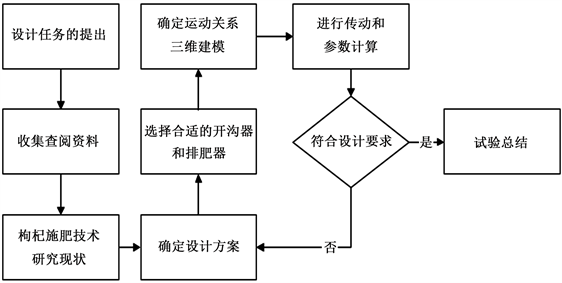Figure 2. Research the technology roadmap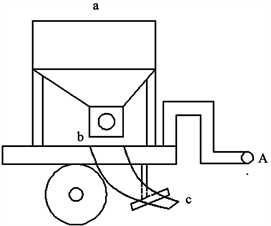Figure 3. Device diagram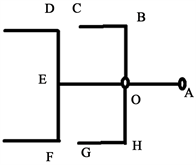(a)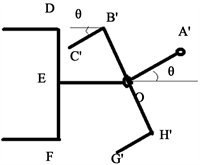(b)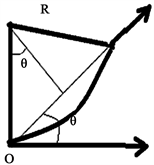(c)

Figure 4. Limit size analysis for suspension parts

${L}_{\text{OE}}\ge {L}_{\mathrm{max}}$

$L={L}_{\text{B′ C ′}}\cdot \mathrm{cos}\theta +{L}_{\text{O B ′}}\cdot \mathrm{sin}\theta$

4. 关键部分的力学分析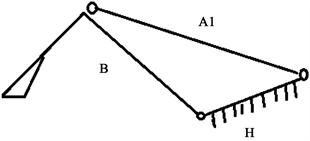(a)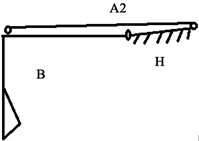(b)

Figure 5. Unilateral analysis of furrow plough on both sides: State (a) and state (b)

$V=\stackrel{˙}{S}=\frac{\partial {S}_{x}}{\partial t}+\frac{\partial {S}_{y}}{\partial t}$

$F=M\cdot a=M\cdot \stackrel{˙}{V}$

${F}_{x}=F\cdot \mathrm{sin}\alpha$

$v=\sqrt{\frac{l\cdot g}{\mathrm{sin}2T}}=\sqrt{\frac{2×9.8}{\mathrm{sin}2×\text{π}÷8}}=4.4\left(\text{m}/\text{s}\right)$

${F}_{S}=9.8{C}_{S}{\delta }^{1.35}\left(1+0.1{\delta }_{c}\right)\left(1-\frac{{90}^{\circ }-\phi }{{180}^{\circ }}\right){e}_{h}$

${C}_{S}$ 为土壤坚实度冲击值，参照沙土地的土壤坚实度冲击值取为6，

${\delta }_{c}$ 为刀片的厚度(mm)，φ为刀片的切削角(˚)， ${e}_{h}$ 为刀片尖角计算系数，带入数据得

${F}_{S}=9.8×6×{4}^{1.35}\left(1+0.1×4\right)\left(1-\frac{{90}^{\circ }-{30}^{\circ }}{{180}^{\circ }}\right)×1=356.29\text{\hspace{0.17em}}\text{N}$

$q=\frac{30}{66~100}=0.3~0.45\text{\hspace{0.17em}}\text{kg}$

$q=\frac{\alpha {F}_{Z}\left({\delta }_{0}+h\right)\gamma }{1000}\left(\text{g}/\text{r}\right)$ (1)

${\delta }_{0}$ 为星轮齿厚(cm)；

h活门开度(cm)；

α为肥料充满系数一般为0.7；

z为星轮槽数设计为6；

γ为肥料的密度，取720 g/L。

$q=\frac{0.7×14.454×6×{\delta }_{0}×720}{1000}=900\text{\hspace{0.17em}}\text{g}/\text{r}$ ${\delta }_{0}=20.59\text{\hspace{0.17em}}\text{cm}$

5. 对关键部分的有限元分析

6. 传动设计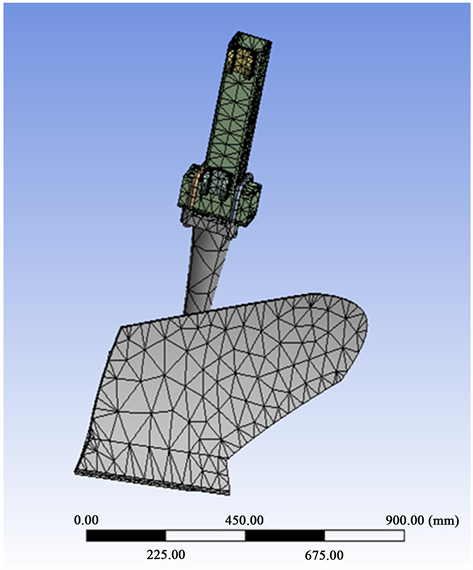Figure 6. Finite element mesh division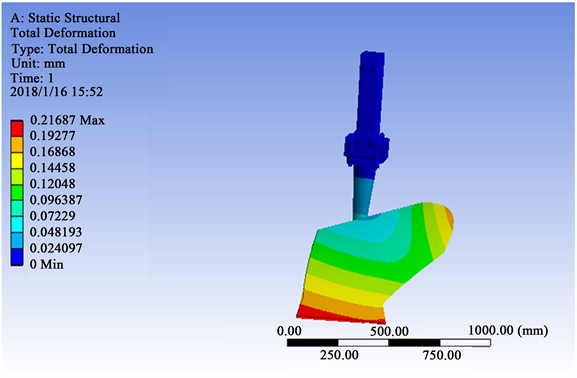Figure 7. Strain diagram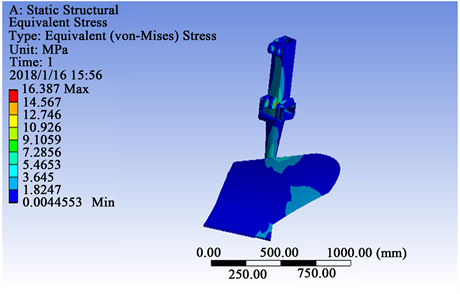Figure 8. Isodynamic map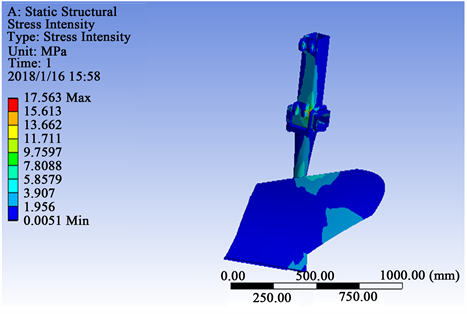Figure 9. Intensity diagram

7. 优势分析

8. 试验验证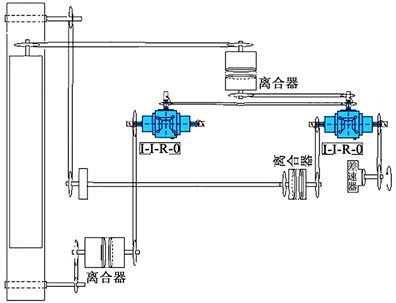Figure 10. Transmission diagram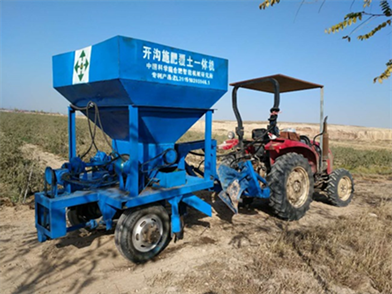Figure 11. Finished product drawing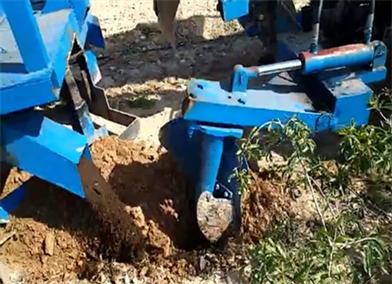Figure 12. Equipment operation condition

2015-2017年宁夏农业综合开发宁夏枸杞产业科技项目“枸杞绿色生产精准作业机械的研制”(编号znnfkj2015-04)；中国科学院STS项目(KFJ-SW-STS-144)。

Mechanical Design for Chinese Wolfberry Organic Fertilizer Deep Homework[J]. 机械工程与技术, 2018, 07(01): 70-79. http://dx.doi.org/10.12677/MET.2018.71009

1. 1. 付宇超, 袁文胜, 纪要. 我国施肥机械化技术现状及问题分析[J]. 农机化研究, 2017(1): 251-255.

2. 2. 王仁祺, 戴铁军. 基于SWOT分析法的宁夏枸杞产业发展战略研究[J]. 安徽农业科学, 2012(28): 413-417.

3. 3. 何义川, 汤智辉, 孟祥金. 2FK-40型果园开沟施肥机的设计与试验[J]. 农机化研究, 2015(12): 201-204.

4. 4. 郭文洋. 精密播种机开沟器的设计与试验研究[J]. 农机化研究, 2014(7): 160-164.

5. 5. 程梅. 果园开沟深施肥机机械结构设计[J]. 安徽农业科学, 2014(23): 400-402.

6. 6. 吉俊宝. 山地柑橘园开沟施肥机的设计与研究[D]: [硕士学位论文]. 武汉: 华中农业大学, 2013.

7. 7. 孙恒、陈作模. 机械原理[M]. 第七版. 北京: 高等教育出版社, 2012.

8. 8. 王延龙. 水稻土条件下的开沟器阻力测试及开沟性能研究[D]: [硕士学位论文]. 南京: 南京农业大学, 2012.

9. 9. 米思米MISUMI. FA工厂自动化用零件[M]. 上海: 米思米(中国)精密机械贸易有限公司, 2015.

10. 10. 濮良贵, 陈国定. 机械设计[M]. 第九版. 北京: 高等教育出版社, 2013.

11. 11. 潘德俊. 自走式枸杞葡萄施肥植保一体机的设计[D]: [硕士学位论文]. 银川: 宁夏大学, 2014.

12. 12. 马爱丽. 基于LS-DYNA果园螺旋开沟机模拟试验研究及其优化设计[D]: [硕士学位论文]. 武汉: 华中农业大学, 2008.

13. NOTES



*通讯作者。